### Estimable Functions

A function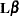is said to be estimable if there exists a linear combination of the expected value of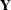, such as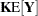, that equals. Since, the definition of estimability implies thatis estimable if there is a matrix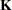such that. Another way of looking at this result is that the rows ofform a generating set from which all estimable functions can be constructed.

The concept of estimability of functions is important in the theory and application of linear models because hypotheses of interest are often expressed as linear combinations of the parameter estimates (for example, hypotheses of equality between parameters,). Since estimability is not related to the particular value of the parameter estimate, but to the row space of, you can test only hypotheses that consist of estimable functions. Further, because estimability is not related to the value of(Searle 1971, p. 181), the choice of the generalized inverse in a situation with rank-deficient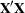matrix is immaterial, since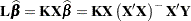whereis invariant to the choice of generalized inverse.is estimable if and only if(see, for example, Searle 1971, p. 185). Ifis of full rank, then the Hermite matrix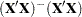is the identity, which implies that all linear functions are estimable in the full-rank case.

See Chapter 15, The Four Types of Estimable Functions, for many details about the various forms of estimable functions in SAS/STAT.